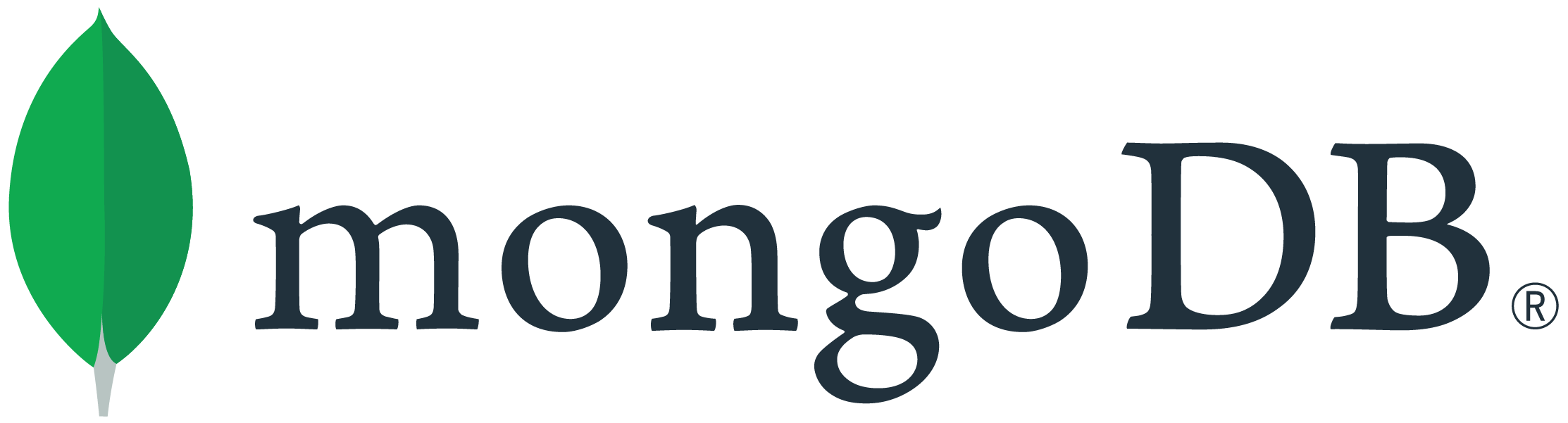# Querying for documents with an ordered sequence of elements in an array

I was playing around with Mongo queries and thought of a case that I cannot find a solution for. Is there an easy way to search for documents that have a specific element sequence in an array? For example, if I have two documents with:
{scores:[1, 2, 3, 4]}
{scores:[4, 3, 2, 1]}

How would I query for a document that has in its ‘scores’ field [2, 3]? From the two documents above, the query should return only the first one.

With simple MongoDB Array operators it is not possible. You can only do exact match or `\$all` or `\$in` which will return both the records. However, I have this solution but it is not as simple as you might have expected:

`````` seq = [2, 3]

db.sequence.find({ \$expr: {
\$in: [
seq,
{ \$map: {
input: { \$range: [0, { \$add: [ { \$size: "\$scores" }, -1 ] }] },
in: [ {\$arrayElemAt: ["\$scores", "\$\$this"]}, {\$arrayElemAt: ["\$scores", { \$add: ["\$\$this", 1 ]}]}]
} }
]
}})
``````

Output:

``````{ "_id" : ObjectId("5c122a2b00746d986abce2f9"), "results" : [ 1, 2, 3, 4 ] }
``````

The above query will not use an index and will perform a collection scan. So we can aggregation pipeline to filter the records which match with the sequence. That will first filter on the list of values that you provide, then project a field checking if the sequence is correct, then filter on that field.

``````seq = [2,3]
db.sequence.aggregate([
{
\$match: {
scores: { \$all: seq }
}
},
{
isSequenceCorrect: {
\$in: [
seq,
{
\$map: {
input: { \$range: [0, { \$add: [{ \$size: "\$scores" }, -1] }] },
in: [{ \$arrayElemAt: ["\$scores", "\$\$this"] }, { \$arrayElemAt: ["\$scores", { \$add: ["\$\$this", 1] }] }]
}
}
]
}
}
},
{ \$match: { isSequenceCorrect: true } }
]);
``````

The output will be:

`````` { "_id" : ObjectId("5c122a2b00746d986abce2f9"), "results" : [ 1, 2, 3, 4 ], "isSequenceCorrect" : true }
``````

Let me know if it helps!

Kanika

1 Like